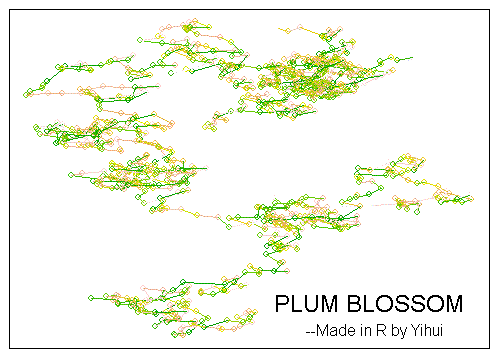# How Can We Survive Such Dining Halls; Plum Blossom & Brownian Motion

### 谢益辉 / 2007-06-13

My poor supper turned out to be a fried steamed bun (mantou) and a cup of yoghourt, because the food in the dining hall was tooooo disgusting.

Dear RUC, do you let live or not let live?! (hai rang bu rang ren huo a?)

Today I suddenly found that the plum blossom was quite similar to Brownian motion @[email protected]!!! Just take a look at this graph (it is in fact a trace for the Brownian motion on the 2-D plane):Below is the source R code (slightly modified):

``````op = par(mar = c(0.5, 0.5, 3, 0.5))
n = 1000
x = apply(d <- matrix(rnorm(2 * n), ncol = 2), 2,
cumsum)
cl = terrain.colors(n)[rank(apply(d, 1, mean))]
plot(x, type = "n", ann = F, axes = F)
title("PLUM BLOSSOM")
mtext("--Made in R by Yihui", side = 3, adj = 1)
box()
for (i in 2:n) {
points(x[i, 1], x[i, 2], col = cl[i])
lines(c(x[i - 1, 1], x[i, 1]), c(x[i - 1, 2], x[i, 2]), col = cl[i])
Sys.sleep(0.01)
}
par(op)
``````